### 奔驰(进口) 奔驰GLS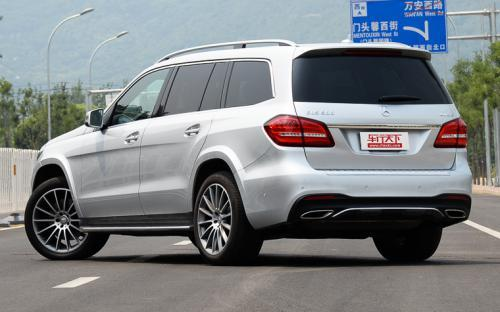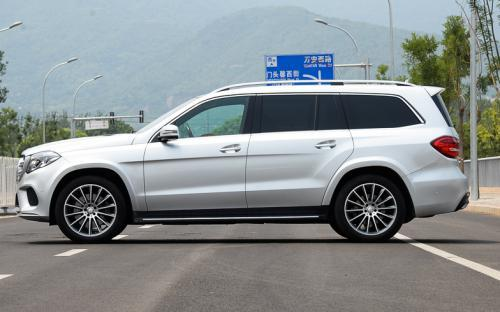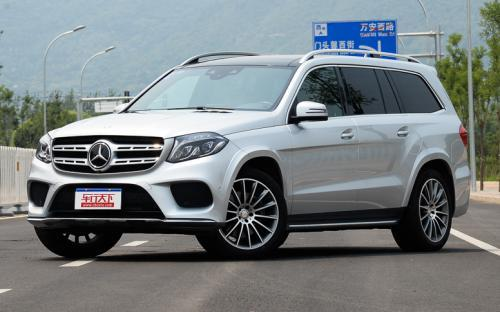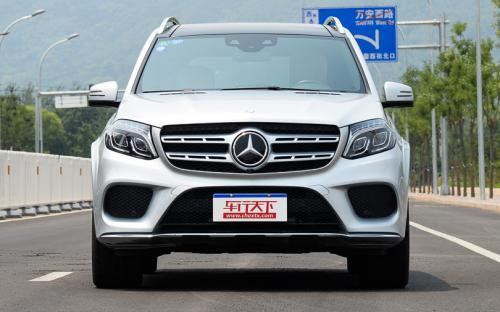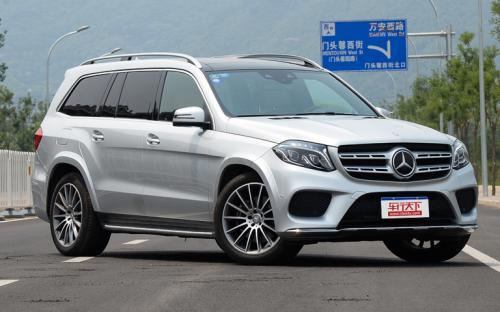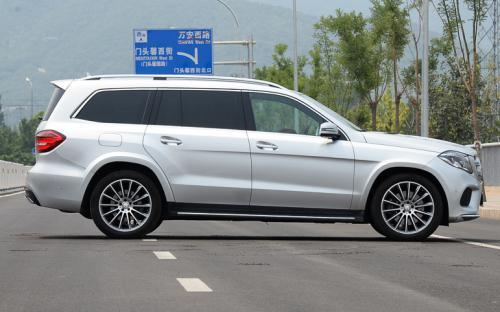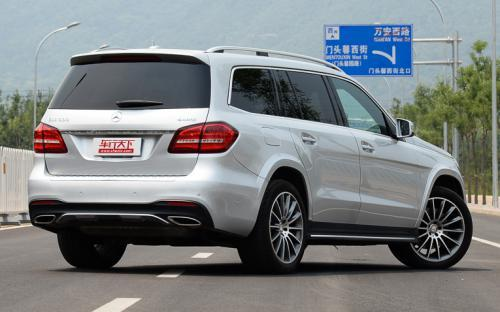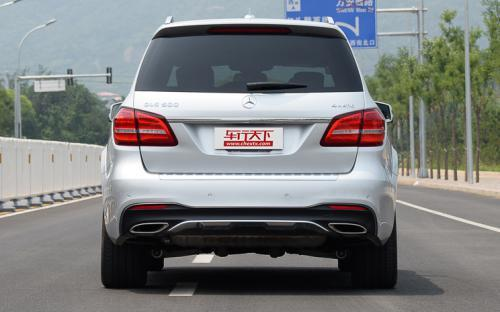0 种颜色可选2016款最低售价：107.60 万元起

5130(mm)1934(mm)1857(mm)##### 配置亮点：
• 胎压监测装置

• ISOFIX儿童座椅接口

• 车身稳定控制(ESC/ESP/DSC等)

• 电动天窗

• 定速巡航

• 后倒车雷达

• 真皮座椅

• GPS导航系统

• 氙气大灯

• 后视镜加热

• 提交
2016款 GLS 500 4MATIC (229张)

奔驰(进口) 奔驰GLS 绕车实拍• 奔驰(进口) 奔驰GLS 在售车型

排量 车型 厂商指导价 本地最低报价 购车工具
3.0L
GLS 350d 4MATIC 柴油 9挡手自一体
107.60万
107.60万

GLS 400 4MATIC动感型 9挡手自一体
115.80万
115.80万

GLS 400 4MATIC豪华型 9挡手自一体
134.30万
GLS 500 4MATIC 9挡手自一体
159.80万
159.80万

4.0L
GLS 350d 4MATIC 柴油 9挡手自一体
107.60万
107.60万

GLS 400 4MATIC动感型 9挡手自一体
115.80万
115.80万

GLS 400 4MATIC豪华型 9挡手自一体
134.30万
GLS 500 4MATIC 9挡手自一体
159.80万
159.80万

奔驰(进口) 奔驰GLS 经销商

查看更多 >>

### 奔驰(进口) 奔驰GLS 动力加速

奔驰GLS 0-100公里加速时间分布在 5.9-8.3秒 属于 高性能级

动力级别 加速时间 车型
运动级(1款)
8.3s
GLS 350d 4MATIC
高性能级(2款)
7.0s
GLS 400 4MATIC动感型GLS 400 4MATIC豪华型
5.9s
GLS 500 4MATIC

奔驰(进口) 奔驰GLS 视频

奔驰(进口) 奔驰GLS 新闻资讯

# 18款奔驰GLS450 高性能全尺寸SUV翘楚

导购 超过13589次关注

2017款奔驰GLS450动力强劲，外观时尚、运动而又不失大气，新款的GLS身材基本是保持不变，依旧保持全尺寸的魁梧和霸气，如果说够狠、够MAN恐怕家族里非G级莫属，但要...

# 18款奔驰GLS450 坐享舒适与奢华

新闻 超过8285次关注

2018款奔驰GLS450动力强劲，外观时尚、运动而又不失大气，新款的GLS身材基本是保持不变，依旧保持全尺寸的魁梧和霸气如果说够狠、够MAN恐怕家族里非G级莫属，但要说...

# 17款奔驰GLS450全域化通行神器

新闻 超过13648次关注

2017款奔驰GLS450动力强劲，外观时尚、运动而又不失大气，新款的GLS身材基本是保持不变，依旧保持全尺寸的魁梧和霸气，如果说够狠、够MAN恐怕家族里非G级莫属，但要...

# 奔驰GLS级正式上市 售价107.6-199.8万元

上市新车 超过8763次关注

2016年3月10日，梅赛德斯-奔驰GLS级正式上市，GLS级是之前GL级的中期改款车型，所以无论外观还是内饰并没有做出大刀阔斧的变化，只是针对细节进行了调整。

# 奔驰GLS级低伪装路试照 GL系列改款更名

评测 超过8473次关注

日前，海外媒体捕获到了一组奔驰GLS级车型的低伪装路试谍照，路试中的车型包含普通版本以及AMG套件版本。关于奔驰GLS级，其就是奔驰现款GL级改款车型，并采用了奔驰...

# 2016年初发布 奔驰新款GLS路试谍照

新闻 超过3434次关注

日前，海外媒体捕获到了一组奔驰新款GLS级车型的路试谍照，GLS级是原GL级车型的最新命名方式）。据悉，这款车预计将于2016年初发布。

猜你喜欢

﻿
• 快速找车
• 选择品牌
• 选择品牌
• A  奥迪
• A  阿斯顿·马丁
• A  阿尔法·罗密欧
• B  宝沃
• B  布加迪
• B  巴博斯
• B  保时捷
• B  宾利
• B  奔驰
• B  宝马
• B  本田
• B  别克
• B  标致
• B  比亚迪
• B  宝骏
• B  北汽制造
• B  北汽新能源
• B  北汽幻速
• B  北汽威旺
• B  北京汽车
• B  奔腾
• B  北汽绅宝
• C  长安
• C  长安商用
• C  长城
• C  昌河
• D  大众
• D  道奇
• D  DS
• D  东南
• D  东风风神
• D  东风风行
• D  东风小康
• D  东风风度
• D  东风
• F  福特
• F  丰田
• F  菲亚特
• F  法拉利
• F  福田
• F  福迪
• F  福汽启腾
• G  观致
• G  广汽传祺
• G  广汽吉奥
• G  GMC
• H  红旗
• H  汉腾汽车
• H  哈弗
• H  哈飞
• H  海格
• H  海马
• H  华颂
• H  黄海
• H  华泰
• H  恒天
• J  吉利汽车
• J  捷豹
• J  Jeep
• J  江淮
• J  江铃
• J  金杯
• J  九龙
• J  金旅
• K  凯翼
• K  凯迪拉克
• K  克莱斯勒
• K  科尼塞克
• K  卡威
• K  开瑞
• L  路虎
• L  林肯
• L  劳斯莱斯
• L  兰博基尼
• L  雷克萨斯
• L  铃木
• L  雷诺
• L  理念
• L  力帆
• L  莲花汽车
• L  猎豹
• L  路特斯
• L  陆风
• M  马自达
• M  MG
• M  MINI
• M  玛莎拉蒂
• M  摩根
• M  迈凯轮
• N  纳智捷
• O  欧宝
• O  讴歌
• O  欧朗
• Q  奇瑞
• Q  起亚
• Q  启辰
• R  日产
• R  荣威
• R  瑞麒
• S  三菱
• S  斯威汽车
• S  萨博
• S  smart
• S  斯柯达
• S  斯巴鲁
• S  思铭
• S  双龙
• S  上汽大通
• S  双环
• T  特斯拉
• T  腾势
• W  沃尔沃
• W  五菱汽车
• W  五十铃
• W  威兹曼
• W  威麟
• X  现代
• X  雪佛兰
• X  雪铁龙
• X  西雅特
• Y  一汽
• Y  英菲尼迪
• Y  英致
• Y  依维柯
• Y  野马汽车
• Y  永源
• Z  众泰
• Z  中华
• Z  中兴
• Z  知豆
• 选择车系
• 选择车系
• 车型对比
• 选择品牌
• 选择品牌
• A  奥迪
• A  阿斯顿·马丁
• A  阿尔法·罗密欧
• B  宝沃
• B  布加迪
• B  巴博斯
• B  保时捷
• B  宾利
• B  奔驰
• B  宝马
• B  本田
• B  别克
• B  标致
• B  比亚迪
• B  宝骏
• B  北汽制造
• B  北汽新能源
• B  北汽幻速
• B  北汽威旺
• B  北京汽车
• B  奔腾
• B  北汽绅宝
• C  长安
• C  长安商用
• C  长城
• C  昌河
• D  大众
• D  道奇
• D  DS
• D  东南
• D  东风风神
• D  东风风行
• D  东风小康
• D  东风风度
• D  东风
• F  福特
• F  丰田
• F  菲亚特
• F  法拉利
• F  福田
• F  福迪
• F  福汽启腾
• G  观致
• G  广汽传祺
• G  广汽吉奥
• G  GMC
• H  红旗
• H  汉腾汽车
• H  哈弗
• H  哈飞
• H  海格
• H  海马
• H  华颂
• H  黄海
• H  华泰
• H  恒天
• J  吉利汽车
• J  捷豹
• J  Jeep
• J  江淮
• J  江铃
• J  金杯
• J  九龙
• J  金旅
• K  凯翼
• K  凯迪拉克
• K  克莱斯勒
• K  科尼塞克
• K  卡威
• K  开瑞
• L  路虎
• L  林肯
• L  劳斯莱斯
• L  兰博基尼
• L  雷克萨斯
• L  铃木
• L  雷诺
• L  理念
• L  力帆
• L  莲花汽车
• L  猎豹
• L  路特斯
• L  陆风
• M  马自达
• M  MG
• M  MINI
• M  玛莎拉蒂
• M  摩根
• M  迈凯轮
• N  纳智捷
• O  欧宝
• O  讴歌
• O  欧朗
• Q  奇瑞
• Q  起亚
• Q  启辰
• R  日产
• R  荣威
• R  瑞麒
• S  三菱
• S  斯威汽车
• S  萨博
• S  smart
• S  斯柯达
• S  斯巴鲁
• S  思铭
• S  双龙
• S  上汽大通
• S  双环
• T  特斯拉
• T  腾势
• W  沃尔沃
• W  五菱汽车
• W  五十铃
• W  威兹曼
• W  威麟
• X  现代
• X  雪佛兰
• X  雪铁龙
• X  西雅特
• Y  一汽
• Y  英菲尼迪
• Y  英致
• Y  依维柯
• Y  野马汽车
• Y  永源
• Z  众泰
• Z  中华
• Z  中兴
• Z  知豆
• 选择车系
• 选择车系
• 选择车型
• 选择车型
• 意见反馈